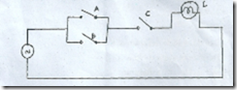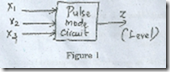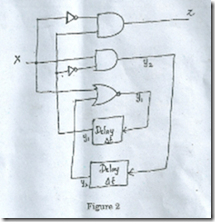### DIGITAL LOGIC CIRCUITS (DLC)–May / June 2012 Question Paper

Anna University

B.E./B.Tech. DEGREE EXAMINATION, MAY/JUNE 2012

Fourth Semester

Electrical and Electronics Engineering

Subject Code : EE 2255/131405/EE 46/EC 1261 A/10133 EE 406/080280029

Subject Name : DIGITAL LOGIC CIRCUITS

(Regulation 2008)

Time : Three hours

Maximum: 100 marks

PARTA—(10x2 =20marks)

1. Express the following switching circuit in binal7 logic notation.2. What is priority encoder?

3. Give the characteristic equation and state diagram of JK flip-flop.

4. What is lockout? How it is avoided? .

5. How does the operation of an asynchronous input differ from that of a synchronous input? .

6.Define flow table in asynchronous sequential circuit.

7.What is a PLA?

8.List the configurable elements in the FPGA architecture.

9.What are the various modeling techniques in HDL?

PARTB—(5x16=80marks)

11. (a) (i) Implement the following Boolean function with NAND – NAND logic. :

Y=AC+ABC+A’BC+AB+D                                                              (6)

(ii) Simplify and implement the following sop function using NOR gates. .

f(A,B,C,D) =  ∑m(0, 1, 4, 5, 10, 11, 14, 15).                                (10)

Or

(b) (i)  Implement the given function using multiplexer

F(x, y, z)= ∑(0, 2, 6, 7).                                                                   (8)

(ii) Implement full subtractor using demuitiplexer.                   (8)

12. (a)(i) Realize SR flip-flop using NOR gates and explain its operation.                                                                                           (8)

(ii) Convert a SR flip-flop into JK flip-flop.                                    (8)

Or

(b) A sequential circuit with 2D FFs A and B and input X and output Y is specified by the following next state and output equations.

A(t+1)=AX+BX .

B(t÷1)=A’X .

Y =(A+B)X’

(i) Draw the logic diagram of the circuit.

(ii) Derive the state table.

(iii) Derive the state diagram.                                                  (16)

13. (a) (i) Design a pulse mode circuit with inputs x1, x2, x3 and output Z as shown in figure 1.(ii) The output should change from O to 1, only for input sequence x1— x2 — x3 occurs while z = O. Also the output z should remain in 1 until x2 occurs Use SR flip-flops for the design.                   (16)

OR

(b) (i) List and explain the steps used for analyzing an asynchronous sequential circuit.                                                                            (8)

(ii) Derive the flow table for the circuit given in the figure 2.   (8)14. (a) Write notes on ROM and its types.                                (16)

Or

(b) (i) A combinational logic circuit is defined by the following function.                                                                                           (10)

f1(a,b,c) =  (O, 1, 6, 7),

f2(a, b, e) =  (2, 3, 5,7)

Implement the circuit with a PAL having three inputs, product terms and two outputs.

(ii) Describe the concept and working of FPGA.                         (6)

15. (a) Explain RTL design using VHDL with the help of example.                                                                                            (16)

Or

(b) Write the VHDL code for mod 6 counter.                             (16)